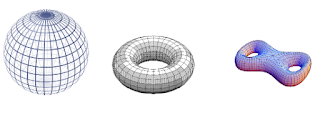#Introduction to Topology -- 1

This page contains a detailed introduction to basic topology. Starting from scratch (required background is just a basic concept of sets), and amplifying motivation from analysis, it first develops standard point-set topology (topological spaces). In passing, some basics of category theory make an informal appearance, used to transparently summarize some conceptually important aspects of the theory, such as initial and final topologies and the reflection into Hausdorff and sober topological spaces. We close with discussion of the basics of topological manifolds and differentiable manifolds, hence of differential topology, laying the foundations for differential geometry.

main page: Introduction to Topology

this chapter: Introduction to Topology 1 – Point-set topology

next chapter: Introduction to Topology 2 – Basic Homotopy Theory

For introduction to more general and abstract homotopy theory see instead at Introduction to Homotopy Theory.

## Total Pageviews

ACTIVE:

### ＰｙｎｄａｎＥＸ - Allocated Industries

Endeavor To Where It Matters! | Economic Calendar by TradingView